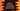# Python find the key of a dictionary with maximum value## Introduction :

In this tutorial, we will learn how to find the key of a dictionary with maximum value in python. We will learn different ways to solve this problem. In these examples, we will use one predefined dictionary.

Python dictionary is used to store key-value pairs. Using the keys, we can access the values. Finding out the largest key is easy, but finding out the largest value and the key associated with it is a little bit tricky.

## Method 1: Find using the maximum key :

In this approach, we will find out the largest value at first. Then, we will find out the key for that value.

``````dict = {"a" : 11, "b" : 2, "c" : 10, "d" : 14}

values = list(dict.values())
keys = list(dict.keys())

m = max(values)

i = values.index(m)

print(keys[i])
``````

Here, dict is the given dictionary. values hold the list of all dictionary values and keys holds the list of all dictionary keys. m is the maximum of all values in the dictionary and i is the index of the maximum value. In the final line, we are printing the key of the maximum value.

## Method 2: Using operator :

Using operator module, it requires only one line :

``````import operator

dict = {"a" : 11, "b" : 2, "c" : 10, "d" : 14}

maxValue = max(dict.items(), key = operator.itemgetter(1))

print(maxValue)
``````

It will print the same output.

## Method 3 : Using max() :

If you don’t want to import any extra module, you can simply use the python max() function. This function is defined as below :

``````max(iterable[, default, key]) -> value
``````

It takes three arguments, one iterable and two more optional arguments default and key.

• iterable: It is an iterable object. This is always required.

• default: This is the default value to return if the first argument iterable is empty. This is an optional argument.

• key: Optional argument. This is a function that is applied to each value of the iterable. Using this function, we can change the way it works.

It returns the maximum item found in the iterable. If the key is provided, it will find the maximum value with natural ordering. Else, it will use the key function to find out the maximum value.

Our goal is to find out the maximum value and its key in a dictionary. We can use the dictionary items() method. It returns a list of all dictionary key, value pairs. Also, we will pass one function lambda i : i) as the key i.e. it will always compare the second element of each iterable element. It will return the item with the largest second value.

``````dict = {"a" : 1, "b" : 2, "c" : 10, "d" : 14}

print(max(dict.items(),key = lambda i : i))
``````

If you execute this program, it will print the below output :

``````('d', 14)
``````

### Method 4: Get only the key using max() :

Method 3, as we have seen above, can be used to get both key-value pairs with the maximum value in a dictionary. But, if you need only the key, you can pass the dictionary as the first argument and dict.get as the second argument. It looks like as below :

``````dict = {"a" : 1, "b" : 2, "c" : 10, "d" : 14}

print(max(dict,key = dict.get))
``````

It will print d as the output.

get() is used to get the value for a key. Using it, max() finds the key with the largest value.